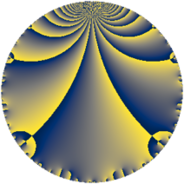# Properties

 Label 45.3.dLevel $45$ Weight $3$ Character orbit 45.d Rep. character $\chi_{45}(44,\cdot)$ Character field $\Q$ Dimension $4$ Newform subspaces $1$ Sturm bound $18$ Trace bound $0$

# Related objects

## Defining parameters

 Level: $$N$$ $$=$$ $$45 = 3^{2} \cdot 5$$ Weight: $$k$$ $$=$$ $$3$$ Character orbit: $$[\chi]$$ $$=$$ 45.d (of order $$2$$ and degree $$1$$) Character conductor: $$\operatorname{cond}(\chi)$$ $$=$$ $$15$$ Character field: $$\Q$$ Newform subspaces: $$1$$ Sturm bound: $$18$$ Trace bound: $$0$$

## Dimensions

The following table gives the dimensions of various subspaces of $$M_{3}(45, [\chi])$$.

Total New Old
Modular forms 16 4 12
Cusp forms 8 4 4
Eisenstein series 8 0 8

## Trace form

 $$4 q + 12 q^{4} + O(q^{10})$$ $$4 q + 12 q^{4} - 28 q^{10} - 76 q^{16} + 80 q^{19} - 44 q^{25} + 104 q^{31} + 112 q^{34} + 28 q^{40} - 56 q^{46} - 308 q^{49} - 72 q^{55} - 88 q^{61} - 116 q^{64} + 504 q^{70} + 240 q^{76} + 56 q^{79} - 112 q^{85} + 504 q^{91} - 224 q^{94} + O(q^{100})$$

## Decomposition of $$S_{3}^{\mathrm{new}}(45, [\chi])$$ into newform subspaces

Label Dim $A$ Field CM Traces $q$-expansion
$a_{2}$ $a_{3}$ $a_{5}$ $a_{7}$
45.3.d.a $4$ $1.226$ $$\Q(\sqrt{-2}, \sqrt{7})$$ None $$0$$ $$0$$ $$0$$ $$0$$ $$q+\beta _{3}q^{2}+3q^{4}+(-\beta _{1}-\beta _{3})q^{5}+\beta _{2}q^{7}+\cdots$$

## Decomposition of $$S_{3}^{\mathrm{old}}(45, [\chi])$$ into lower level spaces

$$S_{3}^{\mathrm{old}}(45, [\chi]) \cong$$ $$S_{3}^{\mathrm{new}}(15, [\chi])$$$$^{\oplus 2}$$